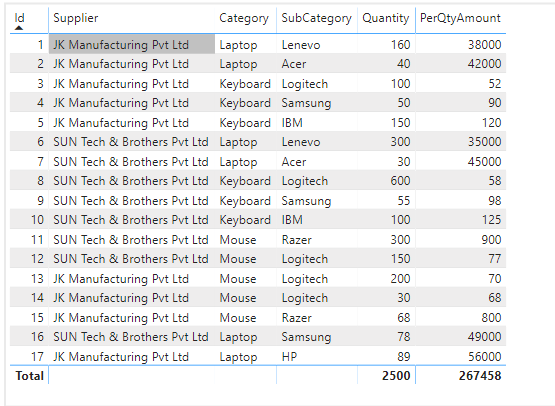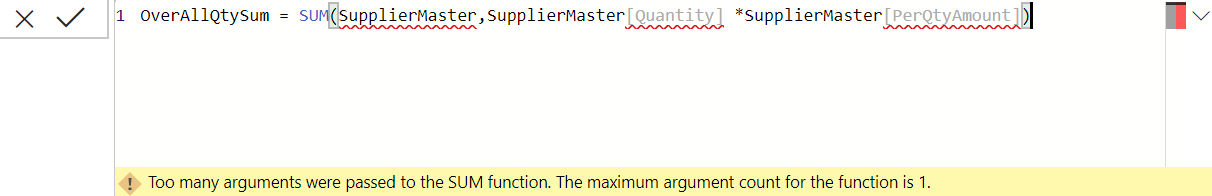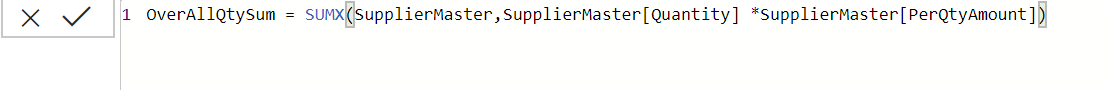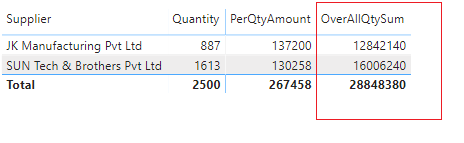Home » SUMX Function DAX

# SUMX Function DAXSUMX Function is a power bi mathematical function in DAX that returns the sum of an expression evaluated for each row in a table. It returns a decimal number.

It goes through a table, row by row to complete the evaluation after filter is applied.

Only the numbers in the column are counted. Blanks, logical values, and text are ignored.

It can be used to evaluate expression over multiple columns as it has the ability to work row by row.

DAX SYNTAX

`SUMX(<table>, <expression>)`

<table> is a base table for which an expression will be evaluated.

<expression> is an expression to be evaluated for each row of the table.

Lets go through an example to see the SUMX function in action.

Following is the sample table which consist a products supplied by the suppliers along with total quantity and per quantity amount by category and subcategory.Now your requirement is to display a overall quantity that will be a quantity * per quantity amount  supplied by individual suppliers .

#### Can we do it using SUM function ?

SUM function operates over a single column of data to aggregate all the data in that single column with the current filters applied. But your requirement is different here as you need to evaluate multiple columns Quantity and PerQtyAmount in a table through row by row to calculate the Overall Quantity by individual supplier.

Lets try to SUM function for this requirement using following cases:

Specify a table name and expression: It gives an error as SUM function accepts only single argument.Specify only expression: It still gives an error as sum function accepts column as reference not an expression.So, if you have a such requirement, in this case you can use SUMX function which can be operated on multiple columns in a table through row by row evaluation in those columns.

Using a SUMX function

```OverAllQtySum =
SUMX(SupplierMaster,
SupplierMaster[Quantity]*SupplierMaster[PerQtyAmount])```Lets commit the measure OverAllQtySum and drag into visual to see the output.

Now you can see the output returned by measure in below screenshot.

It returns the total sum of supplied quantity for each supplier by evaluating the sum for quantity * perQtyAmount row by row through the specified table.